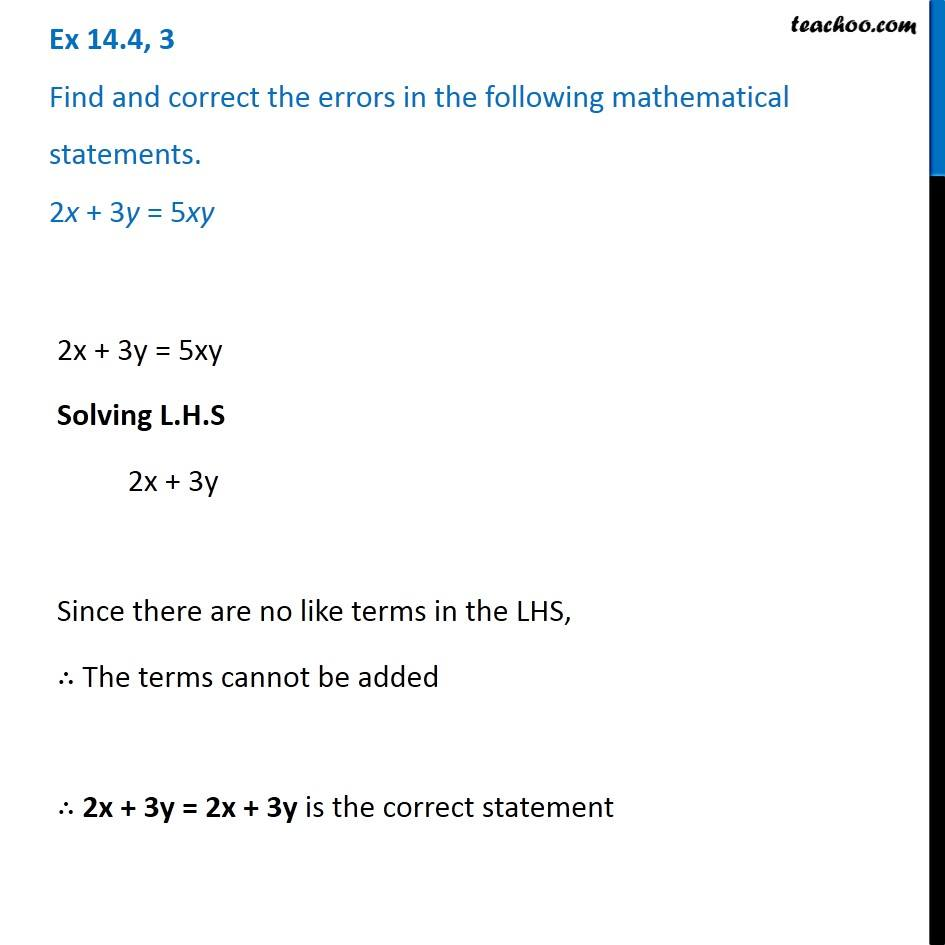Ex 14.4

Chapter 14 Class 8 Factorisation
Serial order wise### Transcript

Ex 14.4, 3 Find and correct the errors in the following mathematical statements. 2x + 3y = 5xy 2x + 3y = 5xy Solving L.H.S 2x + 3y Since there are no like terms in the LHS, ∴ The terms cannot be added ∴ 2x + 3y = 2x + 3y is the correct statement